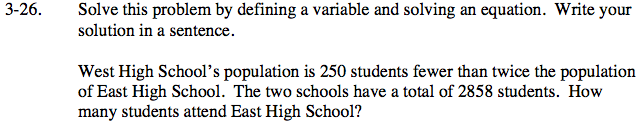### Home > CAAC > Chapter 3 > Lesson 3.1.3 > Problem3-26

3-26.Define a variable.

x = the number of East High School students

Write an expression that defines the number of West High students in terms of the variable.

2x − 250 = the number of West High students

Write an equation using the total population of the two schools.

x + (2x − 250) = 2858

Solve for x.